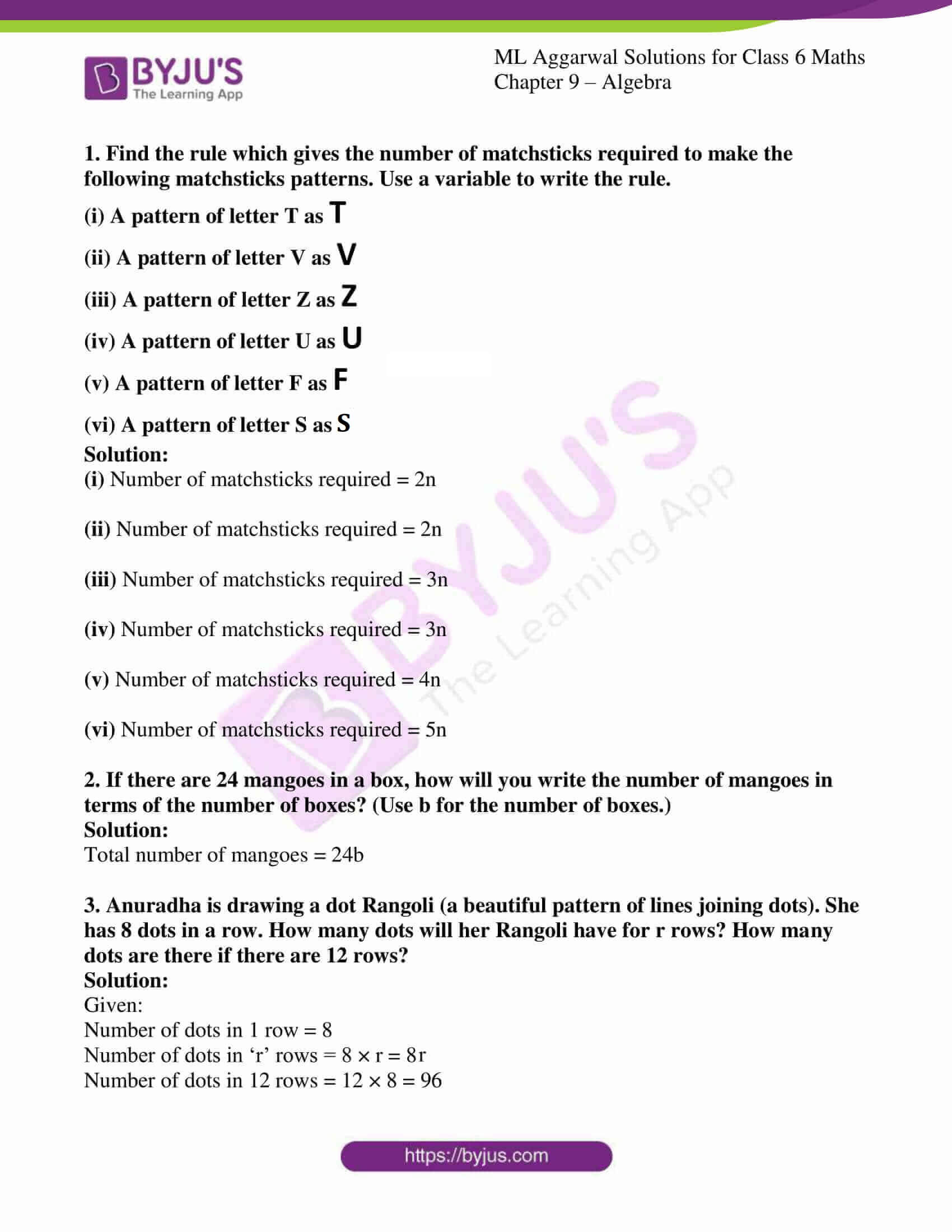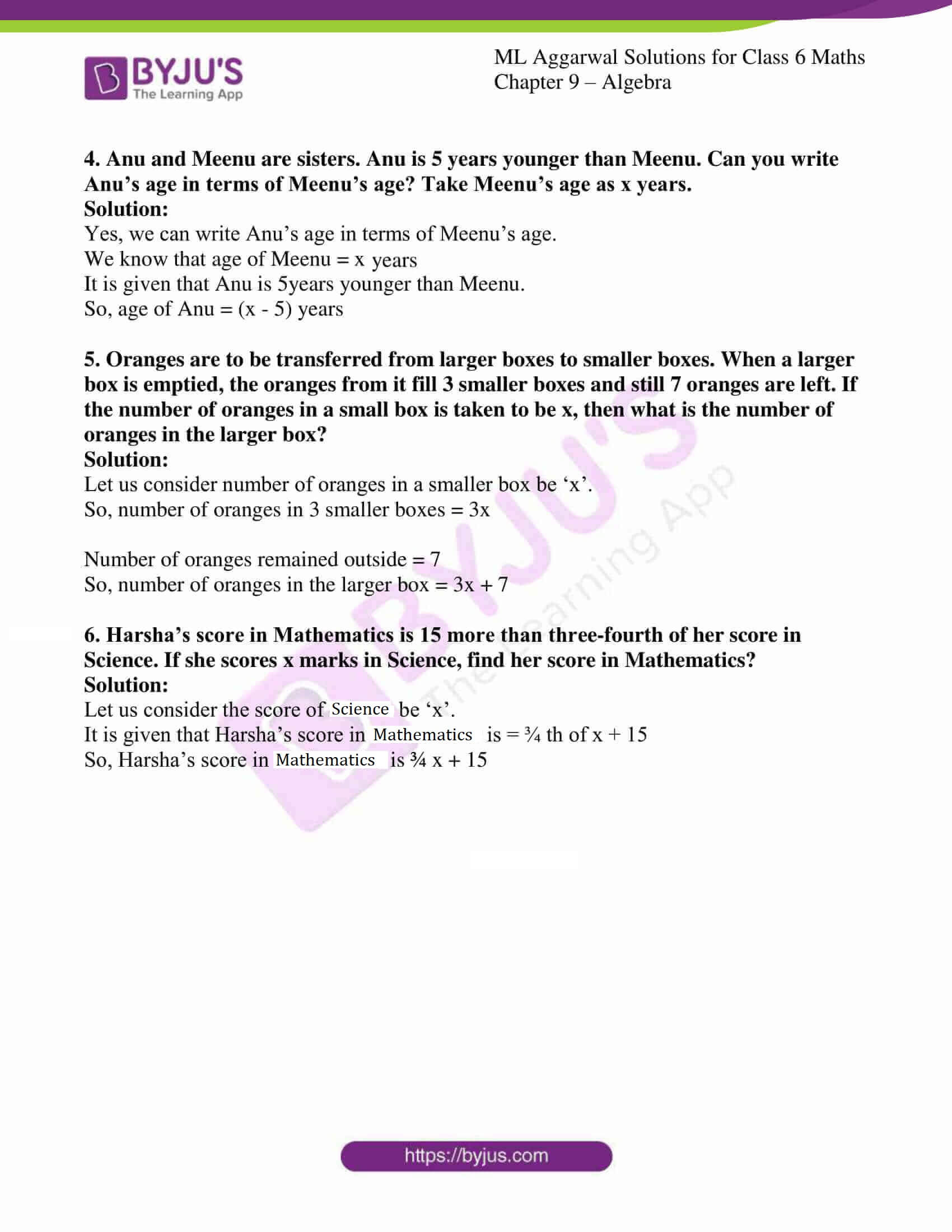# ML Aggarwal Solutions for Class 6 Maths Chapter 9 - Algebra

ML Aggarwal Solutions for Class 6 Maths Chapter 9 – Algebra are provided here to help students understand the concepts clearly and score well in their board exams. This Chapter mainly deals with problems related to algebraic expressions. Students who aim to secure top scores in their examinations can refer to ML Aggarwal Solutions, which is the best reference material prepared by our experts, keeping in mind the latest syllabus. By referring to these solutions, students can follow the correct methodology for solving problems and can also clear their doubts pertaining to any question. Students are advised to practice on a regular basis which yields good results in their exams.

Chapter 9 – Algebra provides solutions to questions related to each and every topic present in this Chapter. Students can refer to ML Aggarwal Class 6 Solutions and easily download the PDF for free, from the links given below and can start practising offline.

## Download the PDF of ML Aggarwal Solutions for Class 6 Maths Chapter 9 – Algebra### Access answers to ML Aggarwal Solutions for Class 6 Maths Chapter 9 – Algebra

1. Find the rule which gives the number of matchsticks required to make the following matchsticks patterns. Use a variable to write the rule.

(i) A pattern of letter T as T

(ii) A pattern of letter V as V

(iii) A pattern of letter Z as Z

(iv) A pattern of letter U as U

(v) A pattern of letter F as F

(vi) A pattern of letter S as S

Solution:

(i) Number of matchsticks required = 2n

(ii) Number of matchsticks required = 2n

(iii) Number of matchsticks required = 3n

(iv) Number of matchsticks required = 3n

(v) Number of matchsticks required = 4n

(vi) Number of matchsticks required = 5n

2. If there are 24 mangoes in a box, how will you write the number of mangoes in terms of the number of boxes? (Use b for the number of boxes.)
Solution:

Total number of mangoes = 24b

3. Anuradha is drawing a dot Rangoli (a beautiful pattern of lines joining dots). She has 8 dots in a row. How many dots will her Rangoli have for r rows? How many dots are there if there are 12 rows?
Solution:

Given:

Number of dots in 1 row = 8

Number of dots in ‘r’ rows = 8 × r = 8r

Number of dots in 12 rows = 12 × 8 = 96

4. Anu and Meenu are sisters. Anu is 5 years younger than Meenu. Can you write Anu’s age in terms of Meenu’s age? Take Meenu’s age as x years.
Solution:

Yes, we can write Anu’s age in terms of Meenu’s age.

We know that age of Meenu = x years

It is given that Anu is 5years younger than Meenu.

So, age of Anu = (x – 5) years

5. Oranges are to be transferred from larger boxes to smaller boxes. When a larger box is emptied, the oranges from it fill 3 smaller boxes and still 7 oranges are left. If the number of oranges in a small box is taken to be x, then what is the number of oranges in the larger box?
Solution:

Let us consider number of oranges in a smaller box be ‘x’.

So, number of oranges in 3 smaller boxes = 3x

Number of oranges remained outside = 7

So, number of oranges in the larger box = 3x + 7

6. Harsha’s score in Mathematics is 15 more than three-fourth of her score in Science. If she scores x marks in Science, find her score in Mathematics?
Solution:

Let us consider the score of Science be ‘x’.

It is given that Harsha’s score in Mathematics is = ¾ th of x + 15

So, Harsha’s score in Mathematics is ¾ x + 15